# Part 5: Trigonometric FunctionsIn this article, we will be examining Year 11 trigonometric functions in more detail and learning to convert between degrees and radians. This opens up many more applications in mathematics and can be incorporated into arcs and circles which is another section we’ll be exploring!

## Year 11 Advanced Mathematics: Trigonometric Functions

Understanding trigonometric functions is an extremely important skill for later topics. By being able to solve trigonometric functions effectively, harder concepts such as bearings, differentiation, etc., become considerably easier to master and solve.

## NESA Syllabus Outcomes

We’re going to look at the following NESA syllabus points:

• Understand the unit circle definition $$sinθ, cosθ$$ and $$tanθ$$ and periodicity using degrees
• Sketch the trigonometric functions in degrees for $$0° \leq x \leq360°$$.
• Define and use radian measure and understand its relationship with degree measure.
• Convert between the two measures, using the fact that $$360°=2\pi$$ radians.
• Recognise and use the exact values of $$sinθ,cosθ$$ and $$tanθ$$ in both degrees and radians for integer multiples of $$\frac{\pi}{6}$$ and $$\frac{\pi}{4}$$.
• Understand the unit circle definition of $$sinθ,cosθ$$ and $$tanθ$$ and periodicity using radians.
• Recognise the graphs of $$y=sinx,y=cosx$$ and $$y=tanx$$ and sketch on extended domains in degrees and radians.
• Derive the formula for arc length, $$l=r\theta$$ and for the area of a sector of a circle, $$A=\frac{1}{2} r^2\theta$$.
• Solve problems involving sector areas, arc lengths and combinations of either areas or lengths.

## Assumed Knowledge

Students should already be familiar with trigonometry with right-angled angles, trigonometric functions of a general angle $$(ASTC)$$, the relationship between trigonometric functions ($$sin\theta,cos\theta$$ and $$tan\theta$$), basic trigonometric identities ($$i.e. sin^2 \theta+cos^2 \theta=1$$) and solving equations with one or more trigonometric functions in degrees.

To begin, let’s get familiar with the concept of radian measure.

Radian measure is a different way of measuring angle size and is passed on the number $$\pi$$. Using radians allows us to solve a wider range of mathematical problems, including differentiation of trigonometric functions, etc. As such, although degrees was previously used, radians will now be the primary format.

The size of an angle in radians is defined by the following ratio:

$$\theta= \frac{Arc \ Length \ PQ}{Radius \ OP}$$

Therefore, when $$\theta=180°$$,

\begin{align*}
180°= \frac{Arc \ Length \ of \ Semicircle}{Radius} \\
= \frac{\pi r}{r} \\
= \pi \end{align*}

Using a similar method, the following formulae can be obtained:
\begin{align*}\pi=180° \\
\frac{\pi}{2}=90° \\
2\pi=360°\end{align*}

Hence, if I was to convert from radians to degrees, I would multiply my angle $$\theta$$ by $$\frac{180°}{\pi}$$.

Inversely, to convert from degrees to radians, I would multiply my angle $$θ$$ by $$\frac{\pi}{180°}$$.

Using these formulae, we can also convert our $$x$$-axis on trigonometric graphs to now be in radian form:

### Example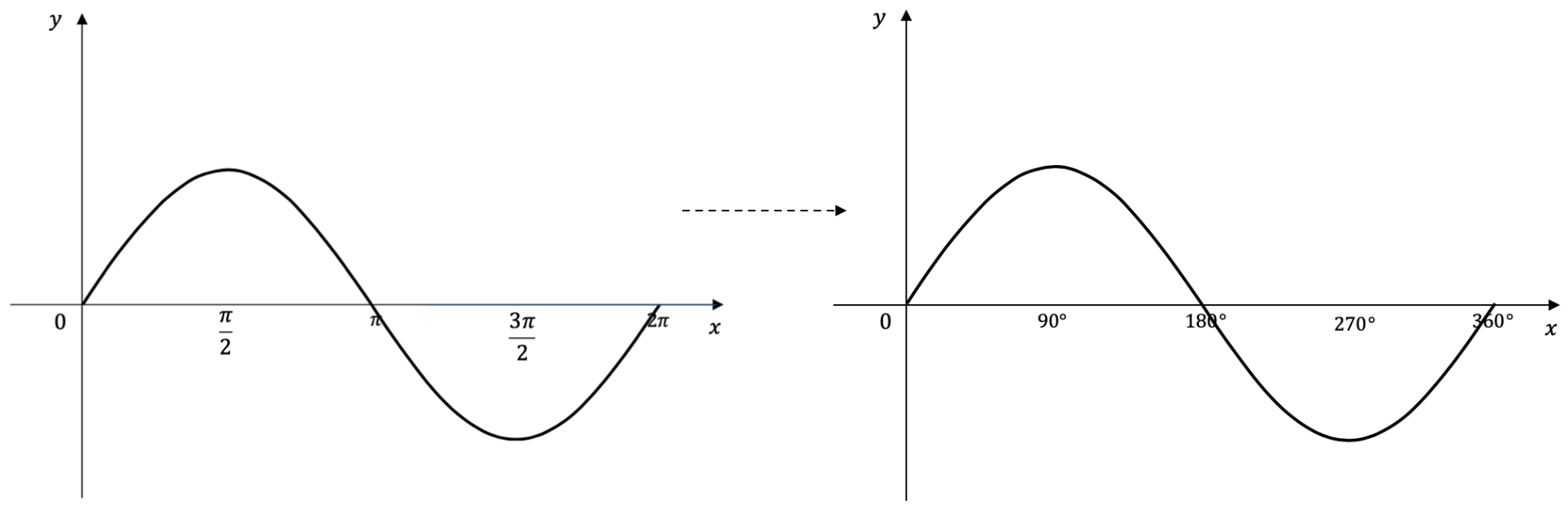## Arcs and Circles

In the diagram on the below, $$AB$$ is an arc of length $$l$$, subtended at centre $$O$$ with angle $$\theta$$.

The formula for arc length is:
$$l=r\theta$$

The formula for the area of sector is:
$$A=\frac{1}{2} r^2 \theta$$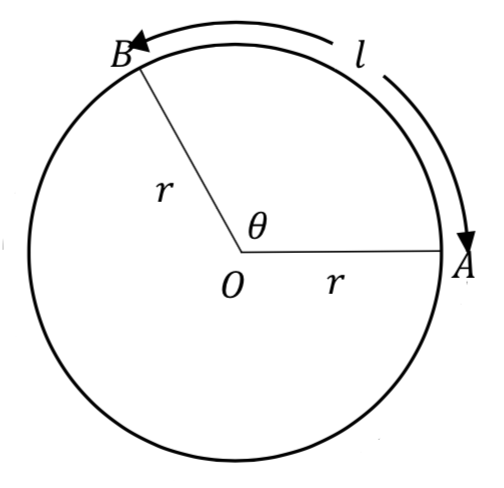In the diagram below, the segment is the blue shaded area between arc $$AB$$ and chord $$AB$$.

The formula for the area of segment is:
$$A=\frac{1}{2} r^2 (\theta-sin\theta)$$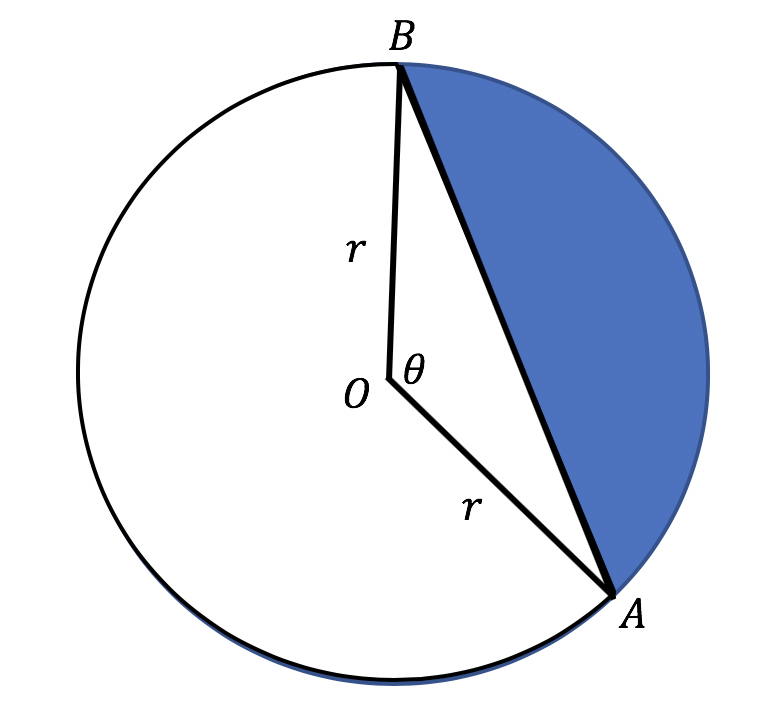### Example

In the diagram below, find:

(a)  The exact area of the sector $$OAB$$.

(b) The exact area of the shaded segment.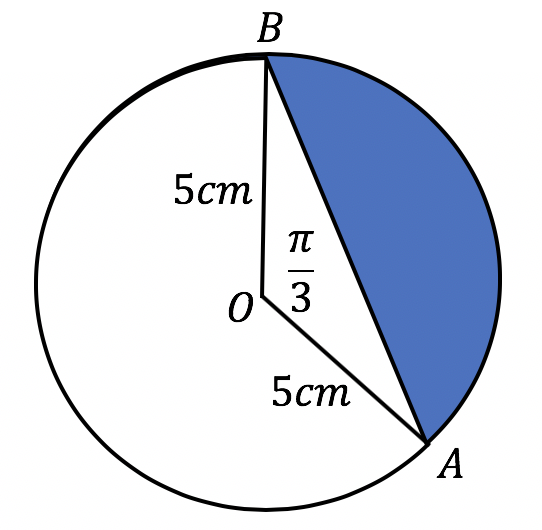### Solution

(a)  Step 1: Re-write the formula for the area of sector:

\begin{align*}
A=\frac{1}{2} r^2 \theta
\end{align*}

Step 2: Substitute values for $$r$$ and $$\theta$$ into the formula:
\begin{align*}
A=\frac{1}{2}\times 5^2 \times sin(\frac{\pi}{3}) \\
A=\frac{25\sqrt3}{4} cm^2
\end{align*}

(b)  Step 1: Re-write the formula for the area of segment:
$$A=\frac{1}{2} r^2 (\theta-sin\theta)$$

Step 2: Substitute values for $$r$$ and $$\theta$$ into the formula:

\begin{align*}
A=\frac{1}{2}\times 5^2 \times (\frac{\pi}{3} – sin(\frac{\pi}{3})) \\
A=\frac{25}{2}(\frac{\pi}{3} – \frac{\sqrt3}{2}) \\
=\frac{25\pi}{6} – \frac{25\sqrt3}{4} cm^2
\end{align*}

## Solving Trigonometric Functions

As discussed above, radians will now be used to solve all the different types of trigonometric equations learnt thus far. This includes questions which involve:

• Using ASTC to Solve Simple Equation
• Trigonometric Identities
• Complementary Angles
• Sine and Cosine Rule

With all these questions, the first step is to convert radians to degrees. However, once you have become proficient in radians and are able to recognize exact values in the radian format, this first step will not be required.

Ideally, with all trigonometry question, the answer should be left in the format of the question. I.e. if the question is in radians, the answer should be in radians.

### Example 1

Solve $$cos⁡(x)=sin⁡(\frac{\pi}{6})$$

### Solution 1

Firstly, we convert our angle from radians to degrees:
$$\frac{\pi}{6}\times \frac{180°}{\pi}= 30°$$,
Substituting this back in;
$$cos⁡(x)=sin⁡(30°)$$
Now we recognize that this question involved complementary angles and use this to convert $$cos⁡(x)$$ into $$sin⁡(90°-x)$$:
\begin{align*} sin⁡(90°-x)=sin⁡(30°) \\
90°-x= 30° \\
x= 60°\end{align*}
Finally, we want to leave our answer in radians as the question was in radians. Hence, we use our formulae to convert our angle from degrees to radians.
\begin{align*}60°\times \frac{\pi}{180°} \\
= \frac{\pi}{3} \\
x= \frac{\pi}{3}\end{align*}

### Example 2

Solve $$sin^2 (x)=\frac{1}{4}$$. Leave your answer in radians.

### Solution 2

Initially, we solve this equation as we would do normally using the ASTC rule:
$$sin^2 (x)=\frac{1}{4}$$

First, we square root both sides;
$$sin⁡(x)= \pm\frac{1}{2}$$
$$sin⁡(x)= \frac{1}{2}$$ or $$sin⁡(x)= \frac{-1}{2}$$

Using the ASTC rule,
If $$sin⁡(x)=\frac{1}{2}$$, $$x$$ must be in the first or second quadrant;
$$x=30°,150°$$
If $$sin⁡(x)=\frac{-1}{2}$$, $$x$$ must be in the third or fourth quadrants;
$$x=210°,330°$$

Therefore, we end up with 4 solutions:
$$x=30°,150°,210°,330°$$

Lastly, we need to convert our angles from degrees to radians:
$$30°\times \frac{\pi}{180°}= \frac{\pi}{6}$$, etc.

Finally, we end up with the following solutions:
$$x= \frac{\pi}{6},\frac{5\pi}{6},\frac{7\pi}{6},\frac{11\pi}{6}$$

## Not seeing the sine of your marks improving?## Concept Check Questions

### Question 1

Express:

(a)  $$\frac{5\pi}{6}$$ in degrees

(b)  $$240°$$ in radians

### Question 2

In the diagram below, find the exact area of the shaded region: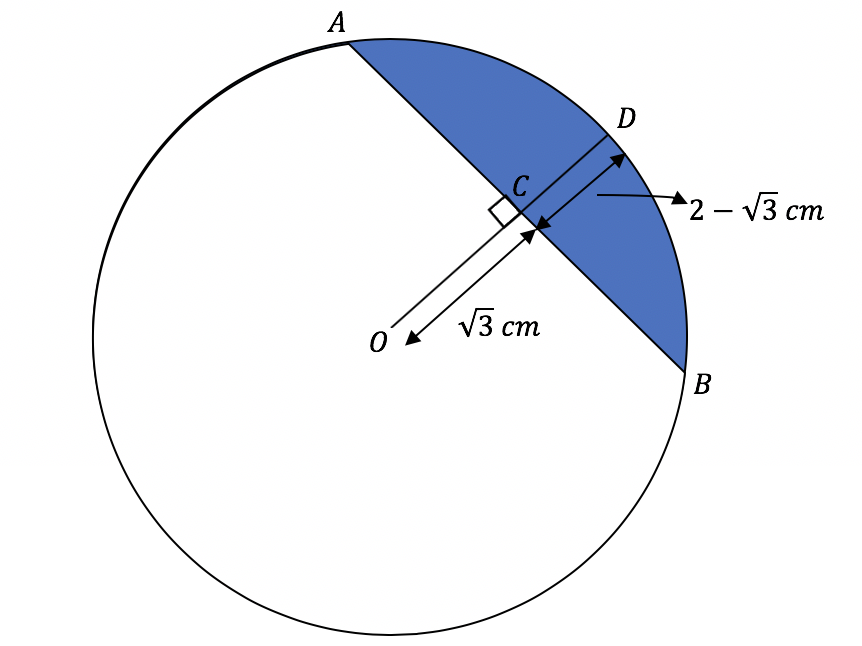### Question 3

Solve for $$x$$ over the domain $$0\leq x \leq 2\pi$$:

(a)  $$sec(x)=2$$

(b)  $$sin^2 x -\frac{3}{4}=0$$

## Concept Check Solutions

### Solution 1

(a)  Substitute in $$\pi=180°$$
$$\frac{5\pi}{6}=\frac{5 \times 180°}{6}=150°$$

(b)  Step 1: Divide $$240°$$ by $$180°$$
$$\frac{240}{180}=\frac{4}{3}$$

Step 2: Use the fact that $$\pi=180°$$
$$240°=\frac{4\pi}{3}$$

### Solution 2:

From the given diagram, the radius $$OD=\sqrt3 + 2 – \sqrt3 = 2cm$$.
$$OA=OB=2cm$$ (equal radii)
$$\triangle OAB$$ is an isosceles triangle ($$2$$ sides equal)

Now, consider $$\triangle OAC$$: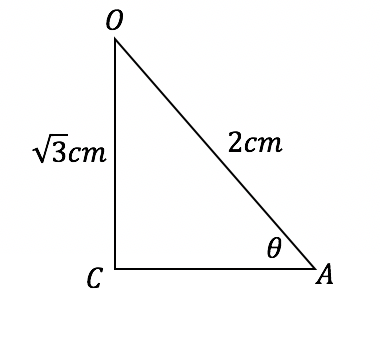Let $$\angle OAC=\theta$$.

Since $$\triangle OAC$$ is right angled at $$C$$, we can use $$sin\theta=\frac{opp}{hyp}$$.

So, $$sin\theta=\frac{OC}{OA}=\frac{\sqrt3}{2} ⟹\theta=sin^{-1}⁡(\frac{\sqrt 3}{2})=\frac{\pi}{3}$$.

And since $$\triangle OAB$$ is isosceles,

$$\angle OAB=\triangle OBA=\frac{\pi}{3}$$ (base angles of isosceles triangles are equal)

Therefore, $$\angle AOB=\pi-2\times \frac{\pi}{3}=\frac{\pi}{3}$$ (angle sum of a triangle)

Using the formula for area of segment, $$A=\frac{1}{2} r^2 (\theta-sin\theta)$$, we can now calculate the exact

area of the shaded region. Substitute $$r=2cm$$ and $$\theta=\frac{\pi}{3}$$ into the formula. We have:

\begin{align*}
A=\frac{1}{2}\times 2^2 (\frac{\pi}{3}-sin(\frac{\pi}{3})) \\
=2\times (\frac{\pi}{3}-\frac{\sqrt3}{2}) \\
A=\frac{2\pi}{3}-\sqrt3 cm^2.
\end{align*}

### Solution 3:

Refer to then diagram below when examining the two solutions: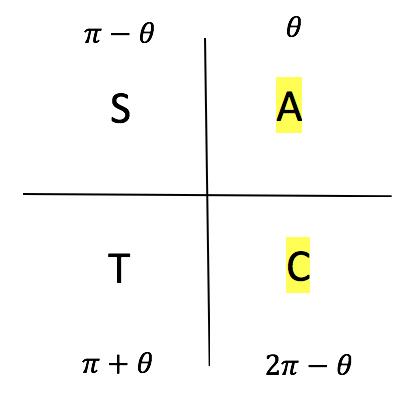(a)   \begin{align*}
secx=2 \\
\frac{1}{cosx}=2 \\
cosx=\frac{1}{2} \\
\end{align*}

Using ASTC, $$cosx \geq 0$$ in $$2$$ regions:

$$\theta$$ and $$2\pi-\theta$$.

$$x=cos^{-1}⁡(\frac{1}{2})$$ $$=\frac{\pi}{3}, 2\pi – \frac{\pi}{3}$$ $$x=\frac{\pi}{3}, \frac{5π}{3}$$

(b)  $$sin^2 x \times – \frac{3}{4}=0$$

$$sin^2 x – \frac{3}{4}=0$$

$$sin^2 x=\frac{3}{4}$$.

Now, take the square root of both sides:

$$sinx=\pm\frac{\sqrt3}{2}$$.

This means we must cover all $$4$$ regions in ASTC:

$$x=sin^{-1}⁡(\frac{\sqrt3}{2})$$ $$=\frac{\pi}{3}, \pi – \frac{\pi}{3}, \pi+\frac{π}{3}, 2\pi – \frac{π}{3}$$ $$x=\frac{π}{3}, \frac{2π}{3}, \frac{4\pi}{3}, \frac{5\pi}{3}$$

## Want to check your progress with Trigonemtric Functions?© Matrix Education and www.matrix.edu.au, 2021. Unauthorised use and/or duplication of this material without express and written permission from this site’s author and/or owner is strictly prohibited. Excerpts and links may be used, provided that full and clear credit is given to Matrix Education and www.matrix.edu.au with appropriate and specific direction to the original content.